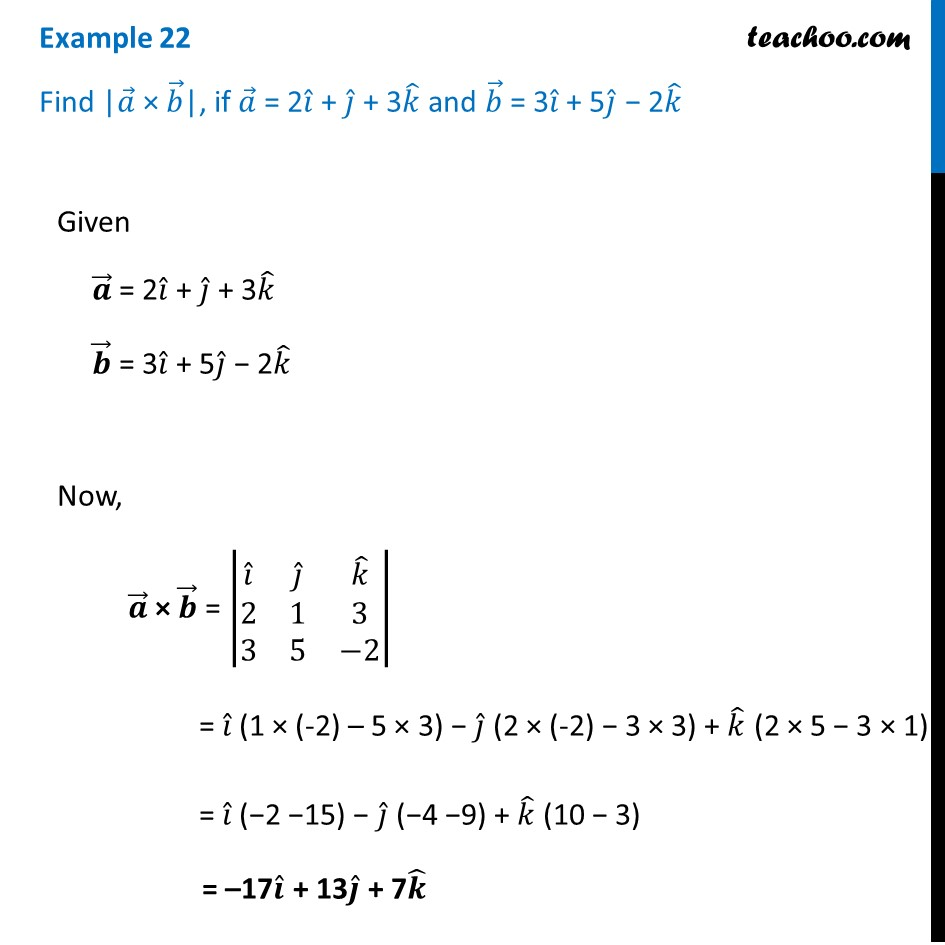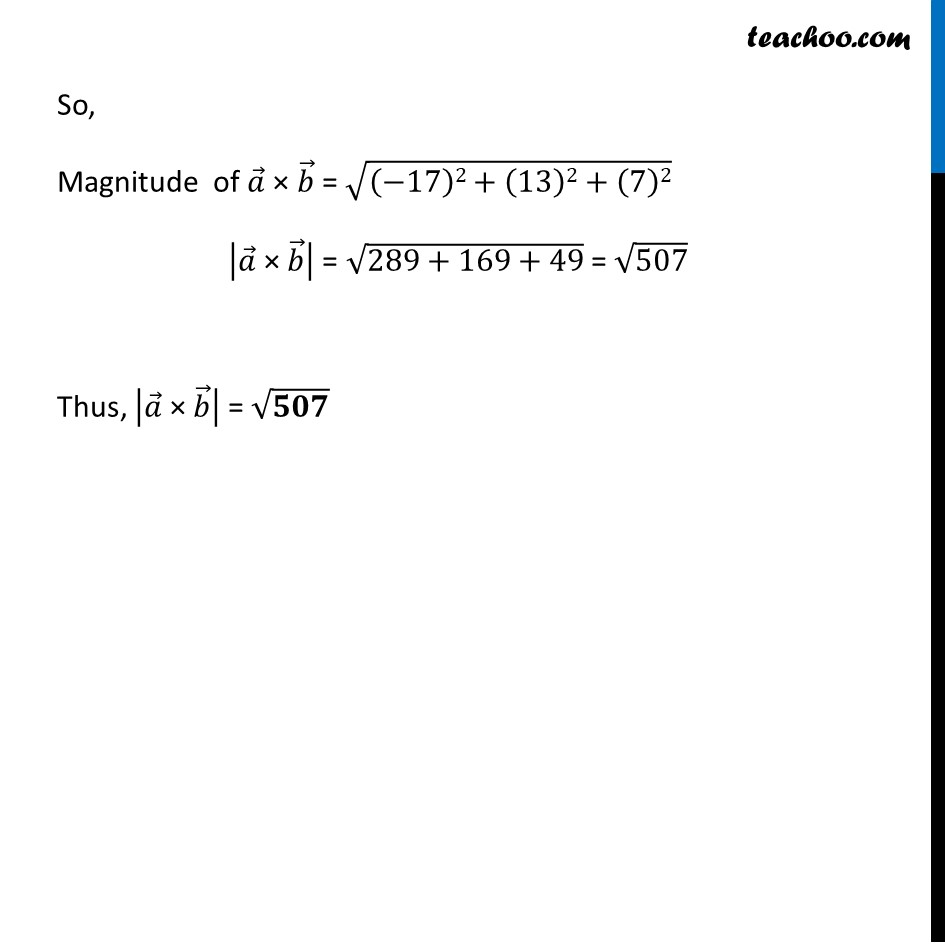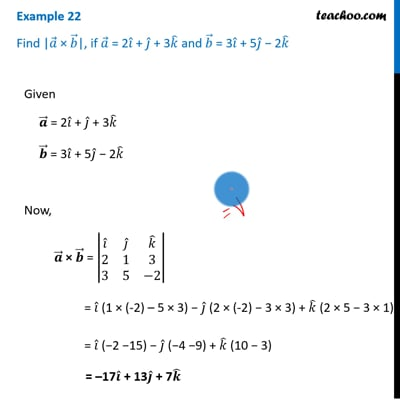Vector product - Defination

Chapter 10 Class 12 Vector Algebra
Concept wiseThis video is only available for Teachoo black users

Introducing your new favourite teacher - Teachoo Black, at only ₹83 per month

### Transcript

Example 22 Find |𝑎 ⃗ × 𝑏 ⃗|, if 𝑎 ⃗ = 2𝑖 ̂ + 𝑗 ̂ + 3𝑘 ̂ and 𝑏 ⃗ = 3𝑖 ̂ + 5𝑗 ̂ − 2𝑘 ̂ Given 𝒂 ⃗ = 2𝑖 ̂ + 𝑗 ̂ + 3𝑘 ̂ 𝒃 ⃗ = 3𝑖 ̂ + 5𝑗 ̂ − 2𝑘 ̂ Now, 𝒂 ⃗ × 𝒃 ⃗ = |■8(𝑖 ̂&𝑗 ̂&𝑘 ̂@2&1&3@3&5&−2)| = 𝑖 ̂ (1 × (-2) – 5 × 3) − 𝑗 ̂ (2 × (-2) − 3 × 3) + 𝑘 ̂ (2 × 5 − 3 × 1) = 𝑖 ̂ (−2 −15) − 𝑗 ̂ (−4 −9) + 𝑘 ̂ (10 − 3) = –17𝒊 ̂ + 13𝒋 ̂ + 7𝒌 ̂ So, Magnitude of 𝑎 ⃗ × 𝑏 ⃗ = √((−17)2+(13)2+(7)2) |𝑎 ⃗" × " 𝑏 ⃗ | = √(289+169+49) = √507 Thus, |𝑎 ⃗" × " 𝑏 ⃗ | = √𝟓𝟎𝟕